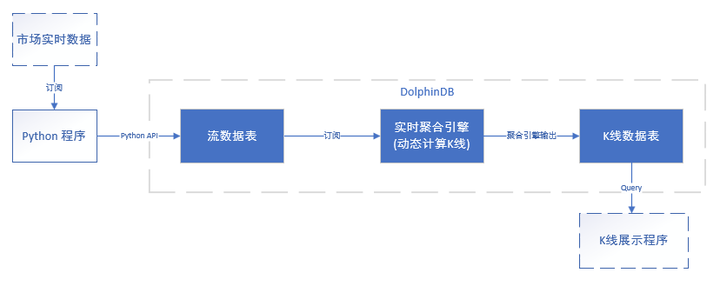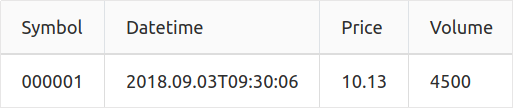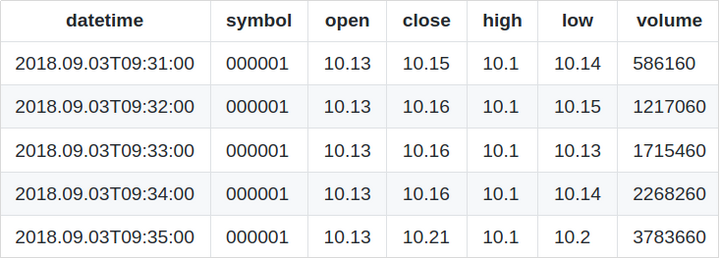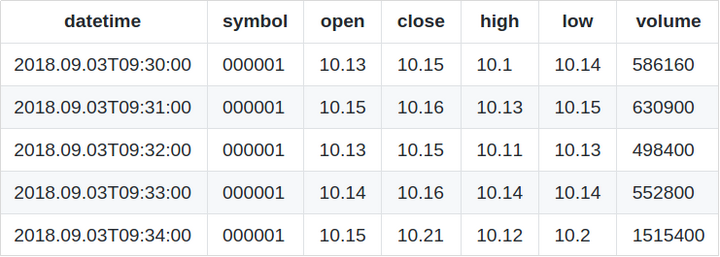### 使用DolphinDB计算K线

DolphinDB提供了功能强大的内存计算引擎，内置时间序列函数，分布式计算以及流数据处理引擎，在众多场景下均可高效的计算K线。本教程将介绍DolphinDB如何通过批量处理和流式处理计算K线。 历史...

DolphinDB提供了功能强大的内存计算引擎，内置时间序列函数，分布式计算以及流数据处理引擎，在众多场景下均可高效的计算K线。本教程将介绍DolphinDB如何通过批量处理和流式处理计算K线。

• 历史数据批量计算K线

• 流式计算K线

1. 历史数据K线计算

1.1 不指定K线窗口的起始时刻，根据数据自动生成K线结果

bar(X,Y)返回X减去X除以Y的余数，一般用于将数据分组。

```date = 09:32m 09:33m 09:45m 09:49m 09:56m 09:56m;
bar(date, 5);```

`[09:30m,09:30m,09:45m,09:45m,09:55m,09:55m]`

```n = 1000000
date = take(2019.11.07 2019.11.08, n)
time = (09:30:00.000 + rand(int(6.5*60*60*1000), n)).sort!()
timestamp = concatDateTime(date, time)
price = 100+cumsum(rand(0.02, n)-0.01)
volume = rand(1000, n)
symbol = rand(`AAPL`FB`AMZN`MSFT, n)
trade = table(symbol, date, time, timestamp, price, volume).sortBy!(`symbol`timestamp)
undef(`date`time`timestamp`price`volume`symbol)```

```barMinutes = 5
OHLC = select first(price) as open, max(price) as high, min(price) as low, last(price) as close, sum(volume) as volume from trade group by symbol, date, bar(time, barMinutes*60*1000) as barStart```

1.2 需要指定K线窗口的起始时刻

```barMinutes = 7
OHLC = select first(price) as open, max(price) as high, min(price) as low, last(price) as close, sum(volume) as volume from trade group by symbol, dailyAlignedBar(timestamp, 09:30:00.000, barMinutes*60*1000) as barStart```

```n = 1000000
date = take(2019.11.07 2019.11.08, n)
time = (09:30:00.000 + rand(2*60*60*1000, n/2)).sort!() join (13:00:00.000 + rand(2*60*60*1000, n/2)).sort!()
timestamp = concatDateTime(date, time)
price = 100+cumsum(rand(0.02, n)-0.01)
volume = rand(1000, n)
symbol = rand(`600519`000001`600000`601766, n)
trade = table(symbol, timestamp, price, volume).sortBy!(`symbol`timestamp)
undef(`date`time`timestamp`price`volume`symbol)```

```barMinutes = 7
sessionsStart=09:30:00.000 13:00:00.000
OHLC = select first(price) as open, max(price) as high, min(price) as low, last(price) as close, sum(volume) as volume from trade group by symbol, dailyAlignedBar(timestamp, sessionsStart, barMinutes*60*1000) as barStart```

```daySession =  08:45:00.000 : 13:45:00.000
nightSession = 15:00:00.000 : 05:00:00.000
n = 1000000
timestamp = rand(concatDateTime(2019.11.06, daySession) .. concatDateTime(2019.11.08, nightSession), n).sort!()
price = 100+cumsum(rand(0.02, n)-0.01)
volume = rand(1000, n)
symbol = rand(`A120001`A120002`A120003`A120004, n)
trade = select * from table(symbol, timestamp, price, volume) where timestamp.time() between daySession or timestamp.time()>=nightSession or timestamp.time()<nightSession order by symbol, timestamp
undef(`timestamp`price`volume`symbol)```

```barMinutes = 7
sessionsStart = [daySession, nightSession]
OHLC = select first(price) as open, max(price) as high, min(price) as low, last(price) as close, sum(volume) as volume from trade group by symbol, dailyAlignedBar(timestamp, sessionsStart, barMinutes*60*1000) as barStart```

1.3 重叠K线窗口：使用wj函数

```n = 1000000
sampleDate = 2019.11.07
symbols = `600519`000001`600000`601766
trade = table(take(sampleDate, n) as date,
(09:30:00.000 + rand(7200000, n/2)).sort!() join (13:00:00.000 + rand(7200000, n/2)).sort!() as time,
rand(symbols, n) as symbol,
100+cumsum(rand(0.02, n)-0.01) as price,
rand(1000, n) as volume)```

`barWindows = table(symbols as symbol).cj(table((09:30:00.000 + 0..23 * 300000).join(13:00:00.000 + 0..23 * 300000) as time))`

```OHLC = wj(barWindows, trade, 0:(30*60*1000),
<[first(price) as open, max(price) as high, min(price) as low, last(price) as close, sum(volume) as volume]>, `symbol`time)```

1.4 使用交易量划分K线窗口

```n = 1000000
sampleDate = 2019.11.07
symbols = `600519`000001`600000`601766
trade = table(take(sampleDate, n) as date,
(09:30:00.000 + rand(7200000, n/2)).sort!() join (13:00:00.000 + rand(7200000, n/2)).sort!() as time,
rand(symbols, n) as symbol,
100+cumsum(rand(0.02, n)-0.01) as price,
rand(1000, n) as volume)

volThreshold = 10000
select first(time) as barStart, first(price) as open, max(price) as high, min(price) as low, last(price) as close
from (select symbol, price, cumsum(volume) as cumvol from trade context by symbol)
group by symbol, bar(cumvol, volThreshold) as volBar```

1.5 使用MapReduce函数加速

(1) 将存于磁盘的原始数据表的元数据载入内存：

```login(`admin, `123456)
db = database("dfs://TAQ")

(2) 在磁盘上创建一个空的数据表，以存放计算结果。以下代码建立一个模板表（model），并根据此模板表的schema在数据库"dfs://TAQ"中创建一个空的 OHLC 表以存放K线计算结果：

```model=select top 1 Symbol, Date, Time.second() as bar, PRICE as open, PRICE as high, PRICE as low, PRICE as close, SIZE as volume from trades where Date=2007.08.01, Symbol=`EBAY
if(existsTable("dfs://TAQ", "OHLC"))
db.dropTable("OHLC")
db.createPartitionedTable(model, `OHLC, `Date`Symbol)```

(3) 使用mr函数计算K线数据，并将结果写入 OHLC 表中：

```def calcOHLC(inputTable){
tmp=select first(PRICE) as open, max(PRICE) as high, min(PRICE) as low, last(PRICE) as close, sum(SIZE) as volume from inputTable where Time.second() between 09:30:00 : 15:59:59 group by Symbol, Date, 09:30:00+bar(Time.second()-09:30:00, 5*60) as bar
return tmp.size()
}
ds = sqlDS(<select Symbol, Date, Time, PRICE, SIZE from trades where Date between 2007.08.01 : 2019.08.01>)
mr(ds, calcOHLC, +)```

2. 实时K线计算

DolphinDB database 中计算实时K线的流程如下图所示：DolphinDB中计算实时K线流程图2.1 使用 Python 接收实时数据，并写入DolphinDB流数据表

• DolphinDB 中建立流数据表
`share streamTable(100:0, `Symbol`Datetime`Price`Volume,[SYMBOL,DATETIME,DOUBLE,INT]) as Trade`

```import dolphindb as ddb
import pandas as pd
import numpy as np
csv_df = pd.DataFrame(csv_data)
s = ddb.session();
#上传DataFrame到DolphinDB，并对Datetime字段做类型转换
s.run("data = select Symbol, datetime(Datetime) as Datetime, Price, Volume from tmpData")

2.2 实时计算K线

• 仅在每次时间窗口结束时触发计算
• 时间窗口完全不重合，例如每隔5分钟计算过去5分钟的K线数据
• 时间窗口部分重合，例如每隔1分钟计算过去5分钟的K线数据
• 在每个时间窗口结束时触发计算，同时在每个时间窗口内数据也会按照一定频率更新
例如每隔1分钟计算过去1分钟的K线数据，但最近1分钟的K线不希望等到窗口结束后再计算。希望每隔1秒钟更新一次

2.2.1 仅在每次时间窗口结束时触发计算

`share streamTable(100:0, `datetime`symbol`open`high`low`close`volume,[DATETIME, SYMBOL, DOUBLE,DOUBLE,DOUBLE,DOUBLE,LONG]) as OHLC`

`tsAggrKline = createTimeSeriesAggregator(name="aggr_kline", windowSize=300, step=300, metrics=<[first(Price),max(Price),min(Price),last(Price),sum(volume)]>, dummyTable=Trade, outputTable=OHLC, timeColumn=`Datetime, keyColumn=`Symbol)`

`tsAggrKline = createTimeSeriesAggregator(name="aggr_kline", windowSize=300, step=60, metrics=<[first(Price),max(Price),min(Price),last(Price),sum(volume)]>, dummyTable=Trade, outputTable=OHLC, timeColumn=`Datetime, keyColumn=`Symbol)`

`subscribeTable(tableName="Trade", actionName="act_tsaggr", offset=0, handler=append!{tsAggrKline}, msgAsTable=true)`### 2.2.2 在每个时间窗口结束触发计算，同时按照一定频率更新计算结果

updateTime参数表示计算的时间间隔，如果没有指定updateTime，只有在每个时间窗口结束时，时间序列聚合引擎才会触发一次计算。但如果指定了updateTime，在以下3种情况下都会触发计算：

• 在每个时间窗口结束时，时间序列聚合引擎会触发一次计算
• 每过updateTime个时间单位，时间序列聚合引擎都会触发一次计算
• 如果数据进入后超过2*updateTime个时间单位（如果2*updateTime不足2秒，则设置为2秒），当前窗口中仍有未计算的数据，时间序列聚合引擎会触发一次计算

• 若将普通的tablestreamTable作为输出表
table与streamTable不会对重复的数据进行写入限制，因此在数据满足触发updateTime的条件而还未满足触发step的条件时，时序聚合引擎会不断向输出表添加同一个time的计算结果，最终得到的输出表就会有大量时间相同的记录，这个结果就没有意义。
• 若将keyedStreamTable作为输出表
keyedStreamTable不允许更新历史记录，也不允许往表中添加key值相同的记录。往表中添加新记录时，系统会自动检查新记录的主键值，如果新纪录的主键值与已有记录的主键值重复时，新纪录不会被写入。在本场景下表现的结果是，在数据还没有满足触发step的条件，但满足触发updateTime的条件时，时序聚合引擎将最近窗口的计算结果写入到输出表，却因为时间相同而被禁止写入，updateTIme参数同样失去了意义。
• 使用keyedTable作为输出表
keyedTable允许更新，往表中添加新记录时，系统会自动检查新记录的主键值，如果新纪录的主键值与已有记录的主键值重复时，会更新表中对应的记录。在本场景下表现的结果是，同一个时间计算结果可能会发生更新。在数据还没有满足触发step的条件，但满足触发updateTime的条件时，计算结果会被修改为根据最近窗口内的数据进行计算的结果，而不是向输出表中添加一条新的记录。直到数据满足触发step的条件时，才会向输出表中添加新的记录。而这个结果才是我们预期想要达到的效果，因此时序聚合引擎要求在使用updateTime参数时，必须使用keyedTable作为输出表。

`share keyedTable(`datetime`Symbol, 100:0, `datetime`Symbol`open`high`low`close`volume,[DATETIME,SYMBOL,DOUBLE,DOUBLE,DOUBLE,DOUBLE,LONG]) as OHLC`

`tsAggrKline = createTimeSeriesAggregator(name="aggr_kline", windowSize=60, step=60, metrics=<[first(Price),max(Price),min(Price),last(Price),sum(volume)]>, dummyTable=Trade, outputTable=OHLC, timeColumn=`Datetime, keyColumn=`Symbol,updateTime=1, useWindowStartTime=true)`

`subscribeTable(tableName="Trade", actionName="act_tsaggr", offset=0, handler=append!{tsAggrKline}, msgAsTable=true)`2.3 在Python中展示K线数据

```import dolphindb as ddb
import pandas as pd
import numpy as np
#设定本地端口20001用于订阅流数据
s.enableStreaming(20001)
def handler(lst):
print(lst)
# 订阅DolphinDB(本机8848端口)上的OHLC流数据表
s.subscribe("127.0.0.1", 8848, handler, "OHLC")```

## 作家榜 »

1.Junxi 73 文章
2.liang.lin 5 文章
3.mhxiang 4 文章
4.wfHuang 3 文章
5.admin 3 文章
6.alex 2 文章
7.丘坤威 1 文章
8.陈枢之 1 文章